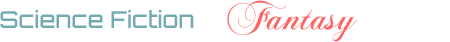## Historical question grades by week

4

(click on this box to dismiss)Q&A for science fiction and fantasy enthusiasts

``````declare @start datetime = '2017-10-08';

with terms as(
select iif(ClosedDate <= CreationDate+3
--and IsClosedAsDuplicate = 0
, 1, 0) closed,
iif(DeletionDate <= CreationDate+3, 1, 0) deleted,

Id PostId
from PostsWithDeleted
where PostTypeId = 1

)

select dateadd(week, datediff(week, @start, CreationDate), @start) week,
/* -- Comment out this line to see all the terms graphed
sum(iif(deleted = 0 and closed = 0 and ((answers > 0 and Score = 0) or Score > 0), 100.0, 0))/count(*) good_not_deleted,
sum(iif(Score > 0, 100.0, 0))/count(*) upvoted,
sum(iif(Score < 0, 100.0, 0))/count(*) downvoted,
sum(iif(Score = 0, 100.0, 0))/count(*) even,
sum(iif(closed = 1, 100.0, 0))/count(*) closed,
sum(iif(deleted = 1, 100.0, 0))/count(*) deleted,
--*/
sum(iif(closed = 1 or Score < 0, 100.0, 0))/count(*) bad,
sum(iif(Score = 0 and answers = 0 and closed = 0, 100.0, 0))/count(*) neutral,
sum(iif(closed = 0 and ((answers > 0 and Score = 0) or Score > 0), 100.0, 0))/count(*) good

from terms
join PostsWithDeleted
on Id = PostId
where PostTypeId = 1
group by dateadd(week, datediff(week, @start, CreationDate), @start)
order by dateadd(week, datediff(week, @start, CreationDate), @start)``````

### Enter Parameters

Options:
-Hold tight while we fetch your results
:records returned in :time ms:cached